# Understanding IEC Aerodynamic Noise Prediction for Control Valves

Floyd D. Jury
Technical Consultant, Fisher Controls International, Inc.

In 1995, the International Electrotechnical Commission (IEC) released International Standard IEC 534-8-3 which is designed to calculate a sound pressure level for aerodynamic noise pro-duced by a control valve. This internationally approved and accepted standard is important because it provides a method for comparing one control valve to another on a common basis. This frees the end user from the burden of deciding which control valve vendor has the best proprietary noise prediction method. An additional advantage of the standard is that all government regulatory bodies will accept the resulting noise calculations.

Although the standard is an easy-to-use document for making aerodynamic noise calculations, it is not a good learning tool. It has little explanation and many equations with little apparent continuity. This Technical Monograph (TM) is designed to provide the user of the IEC standard with a fundamental understanding of the theory behind the standard.

At first glance, IEC 534-8-3 (hereafter referred to as "the standard") would probably lead one to believe that it is just a jumbled mass of empirical equations. Actually, the standard is based on a combination of fundamental theory from the academic fields of thermodynamics and acoustics. The basic equations which are developed from this theory are then modified by experimental results.

The IEC standard basically outlines a five step procedure for calculating noise. While the details and equations of each step often change with the flow regime involved, the general principle does not. The five steps are as follows:

# Step 1

It’s the mechanical power resident in the fluid at the vena contracta of the valve which gets converted to noise. So, the first step is to determine how much mechanical power is resident in the flow stream at the vena contracta.

# Step 2

Most of the mechanical power at the vena contracta is converted to heat, but some of it is converted to noise. Empirically derived acoustic efficiency factors are used to determine how much noise power is generated downstream of the valve.

# Step 3

Once we know what the sound power is in the flowing medium we can use conventional theory to convert this sound power to sound pressure level in the fluid downstream of the valve.

# Step 4

In order to determine how much of the sound pressure level gets transmitted through the pipe wall to the outside air, we must deal with the transmission losses involved in the passage of the sound through the pipe wall. As we shall see, these transmission losses depend upon many different properties of both the fluid and the pipe. The sound pressure level immediately outside the pipe wall is then converted to an A-weighted sound pressure level.

# Step 5

Finally, standard acoustic theory is used to deter-mine how much of this sound pressure level gets transmitted to a hypothetical observer located at the standard location, which is one meter downstream from the valve and one meter away from the outer surface of the pipe.

Understanding these five steps, which are always the same for every application, provides the continuity needed to success-fully work through the standard for any given valve noise application. Let’s turn now to developing a clearer under-standing of what exactly is involved in each of these steps.

# Step 1 - Determine the stream power at the vena contracta.

The noise generated downstream of the valve comes from the dissipation of mechanical energy in the fluid stream at the vena contracta of the valve. We can determine this mechanical energy by using the common equation for calculating kinetic energy of any moving mass. This equation from basic physics is simply one-half the mass times the square of the velocity of the mass.

Since we are interested more in power than we are in energy, we need only recall that power is the time rate of energy. Thus, if we use the mass flow rate in this equation instead of the mass, we will be able to calculate the mechanical power of the fluid stream at the vena contracta, just before any dissipation to noise occurs; i.e.,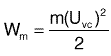Stream power of the mass flow, Watts

The energy equation from the First Law of Thermodynamics provides a convenient way to calculate the velocity of the fluid stream at the vena contracta. Several assumptions are made to further the calculation. For example, it is assumed that there is no elevation change between the vena contracta and the valve outlet (or if there is, it is negligible).

Because the change from the inlet conditions to the vena contracta takes place too rapidly for any significant heat trans-fer, we can safely assume this to be an adiabatic process. There is no work done by the fluid on anything outside the system, and finally, to simplify the calculation, we can assume the fluid velocity at the inlet to the valve is negligible compared to the velocity at the vena contracta. This is a standard assumption in many thermodynamic problems.

It is normally a lot easier to deal with pressures and specific heats, etc. than to deal with enthalpy. This transition can be accomplished if we make some additional assumptions. It is common in many thermodynamic problems to assume that real gases can be represented by the ideal or perfect gas law. This assumption is well within the normal engineering accuracy domain. In addition, it is common to assume that the specific heats are constant over the process. Finally, it is assumed that the process from the valve inlet to the vena contracta is a reversible process which we have already assumed to be adiabatic. Isentropic is a name given to reversible adiabatic pro-cesses.

# Step 2: Convert to noise power at the valve outlet.As the fluid expands from the vena contracta into the down-stream piping, some of the mechanical power at the vena contracta is converted into turbulent noise. This conversion to noise is an extremely complex process which is very difficult to accurately determine in an analytical manner. For this reason, experimentally determined acoustic efficiency factors are used.

In general, the acoustic power developed downstream of the vena contracta is determined by multiplying the vena contracta stream power by an experimentally determined efficiency factor as follows;

NOTE: Low acoustic efficiency is desirable. This means less stream power is converted to noise.

# Step 3: Determine Sound Pressure Level In The Downstream Flow

The noise standard gives a definition of different flow regimes in equation form, but Figure 1 gives a better visual description of these flow regimes. Since a single acoustic efficiency factor cannot be developed that will accurately cover the entire range of possible flow conditions, a separate acoustic efficiency factor is determined for each flow regime. In general, these acoustic efficiency factors are dependent primarily upon Mach number and valve recovery characteristics.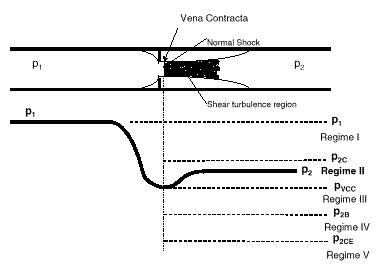Figure 1: Flow regimes

The actual noise production mechanisms present in a control valve are complex enough to give even the Phd’s a headache trying to think about them. No matter. It’s not really important that we understand the precise mechanisms involved as long as we can have a basic appreciation for what is happening.

There are primarily two principle noise mechanisms. In flow regimes 1-3 the primary noise mechanism is due to the turbulence downstream of the vena contracta. This is called, "Turbulent shear flow." As the flow gets more intense due to the higher pressure drop across the valve and we begin the move to higher flow regimes (3-5), the normal shock begins to move further downstream and starts to break up into several smaller shock cells. From each of these shock cells, shock waves are formed which travel downstream at some angle with the centerline. These shock waves bounce off the pipe wall and are reflected across the pipeline to reflect off the opposite wall. As these reflected waves go bouncing down the pipeline, they pass through the area of turbulence creating noise energy and imparting vibrations to the pipe wall as they go. This is called, "Shock-turbulence interaction."

In the final flow regime (V), the noise generated is no longer a function of pressure. This is called the region of constant efficiency.

It is worth noting that the majority of valve applications fall into flow regimes I or II.

# Step 4: Determine the A-Weighted Sound Pressure Level Outside PipeSince our ultimate goal at the observer’s ear is sound pressure level rather than sound power level, we need to make the con-version from power level to pressure level for the noise in the downstream gas. First, we need to know the actual pressure disturbance (pd) which occurs in the downstream gas as a result of the noise power we have calculated. This can be easily obtained from the basic acoustics equation relating sound power and sound pressure shown below.

Theoretically, the noise power generated in the fluid radiates outward in all directions; however, in the usual control valve, little noise travels through the wall of the valve. The noise of interest is only that which travels downstream of the valve inside the pipe, and then escapes through the wall of the pipe.

Consequently, the assumption is made that only one-fourth of the total power calculated is directed downstream and distributed over only a portion of a spherical surface which is equal to the cross sectional area of the pipe. Once we know the actual pressure disturbance (p d) due to thedownstream noise, we can compare this to the standard Pres-sure reference for the threshold of hearing (po= 2 x 10-5Pa) to determine the sound pressure level using the following relationship

So far, this has only determined the sound pressure level in the gaseous fluid inside the pipe. Noise generated inside the pipe is of little concern until it gets outside the pipe. To do this, the noise energy inside the fluid stream must cause the pipe wall to vibrate so as to disturb the surrounding air and produce sound waves that will impact on an observer.

As the pressure waves go spiralling down the pipeline and bouncing off the pipe walls, they impart some energy to the pipe wall which causes it to vibrate. As the pipe wall vibrates, it disturbs the air layer which is in contact with the outside surface of the pipe. These air pressure disturbances at the pipe wall, of course, result in a sound pressure level at this location. Not all of the sound inside of the pipe gets outside the pipe, however. Some is lost in the transmission through the pipe wall.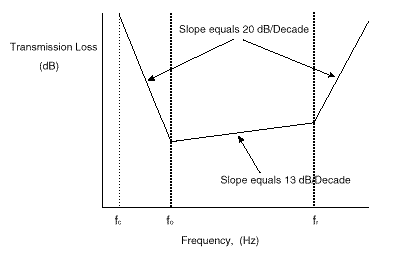Figure 2: Pipe Transmission Loss Spectrum

Determining the sound transmission loss through the pipe wall is a very complicated process and only a

handful of people in the world really understand how it works. A simplistic state-met can be made that a statistical approach is used to perform an energy balance; i.e., energy in equals energy out. The devil is in the details of course, and if you wish to delve into those details you will find them documented in a paper by Dr. A.C. Fagerlund and Dr. D.C. Chou

The transmission losses through the pipe are a function of the downstream fluid properties as well as the pipe properties. As a result, the transmission loss varies with frequency of the noise as shown in Figure 2. For typical valve piping systems, the slopes of this transmission loss curve stay the same. Thus, there are only two critical parameters which completely define this transmission loss spectrum for a given situation, and they are the first-coincidence frequency (fo) and the ring frequency (f r). The first step then in determining the transmission loss for a specific situation is to determine these two frequencies for the given conditions. There is actually a third parameter involved in this spectrum

(i.e., the cut-off frequency fc); however, this parameter is not invoked in the standard. It is included here only for the sake of completeness. These three frequencies always maintain the same relationship to each other as follows.

f c < f o < f r where

f c = Cutoff frequency
f o = First coincidence pipe frequency
f r = Ring frequency

# Cutoff Frequency

Recall that frequency (f) is inversely proportional to wave length (l). Thus, as the frequency gets lower, the wavelength gets longer. When the wavelength is equal to (or longer than) the diameter of the pipe, the whole wave (or some portion thereof) is able to travel down the pipe as a simple plane wave. In this case there is no component of the wave which can exert any pressure fluctuation against the pipe wall and cause any vibration. Thus, for frequencies at (or below) the cut-off frequency, there is no way for the noise inside the pipe to get transmitted outside the pipe wall. In other words, the pipe transmission "loss" is infinite and we don’t need to worry about any noise at frequencies below the cut-off frequency.

# First Coincidence Pipe Frequency

At frequencies above the cut-off frequency, the wavelengths are shorter than the diameter of the pipe. Consequently, the waves tend to travel down the pipeline at different angles with respect to the centerline of the pipe. This means that a particular wave will only travel so far before it bounces off the pipe wall and imparting some energy to the pipe wall in the process.

As the wave bounces off one wall, it is reflected across the diameter of the pipe to bounce off the opposite wall and so on as the wave moves down the pipeline in a spiralling fashion. As a result of the energy transmitted to the pipe by the pressure wave in the fluid, a corresponding stress wave is set up in the pipe wall. This stress wave in the pipe wall spirals down the pipeline in concert with the spiralling pressure wave in the fluid.

If a fly was sitting on the exterior surface of the pipe, it might very well be able to feel the undulations of the pipe surface as the wave goes travelling past. The frequency of these undulations, of course, would depend upon the frequency of the sound wave in the fluid which causes these pipe wall undulations. The undulations which the fly would experience are not due to bending of the whole pipe, but merely localised flexing of the pipe wall as the stress wave travels down the pipe.

Because of the material and geometric properties of the pipe, there will be some "natural" frequency of the pipe wall where the pipe will tend to go into a "resonant" mode. As a result, it takes less energy to excite the pipe at this frequency. Conversely, a given amount of exciting energy will produce a greater amount of vibration amplitude at this frequency. Of course, there are higher frequency harmonics of this frequency as well, but the majority of the energy transmission will occur at this "first" or fundamental frequency.

When the frequency of the spiralling pressure wave in the fluid (and the concurrent stress wave in the pipe) exactly "coincides" with the fundamental resonant frequency of the pipe, we will generate the greatest amplitude of pipe wall vibration from this particular stress wave. This frequency is called the "first coincidence pipe frequency."

Since the pipe wall amplitude is greater at this frequency, a greater amount of noise will be transmitted through the pipe wall at this frequency. Another way of stating this is that the transmission "loss" will be lower at this frequency.

# Ring FrequencyFigure 3: Ring Section of Pipe

Imagine a "ring" section of the downstream pipe as shown in Figure 3. Image further that the applied force shown in the figure is a sinusoidal force created by the effect of the fluid sound pressure waves continuously bouncing off the wall of the pipe at some frequency determined by the frequency of the sound wave. This will cause a corresponding sinusoidal stress wave to travel around the circumference of the pipe.

As this stress wave continues to travel on around the ring back to its starting point, the stress wave arriving back at the starting point will either add to or subtract from the stresses being generated by the next wave striking the pipe wall.

When the distance between wave peaks (wavelength) is exactly equal to the circumference of the pipe, the first stress wave which arrives back at the starting point will be "in phase" with the stress wave created by the following wave. This, of course, will result in a resonant condition which greatly increases the amplitude of the pipe wall motion. The frequency where this "in-phase" reinforcement occurs is called the "ring frequency" and it will occur when the wave length of the stress wave is exactly equal to the circumference of the pipe.

# Peak Frequency of the Noise

Since we have already been given the pipe transmission loss spectrum, we only need to know where the frequency of the noise falls with respect to this spectrum so we can determine how much of the generated noise will get transmitted through the pipe wall to become noise in the observer’s environment.

Aerodynamic noise generated in a control valve has been experimentally shown to produce a noise spectrum which is essentially shaped like a haystack as illustrated in Figure 4. The peak noise level of this spectrum occurs at a frequency called, logically enough, the "peak frequency (f p)."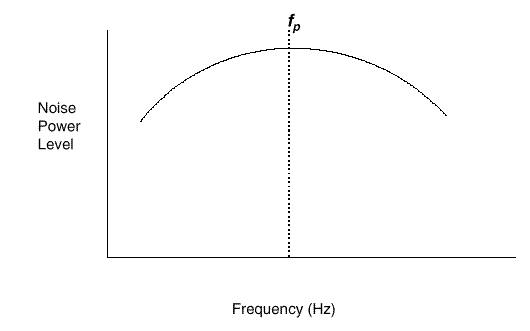Figure 4: Peak Frequency

Strouhal, an early experimenter in turbulent flow, discovered that the tone frequency of turbulent noise could be given by the equation shown here.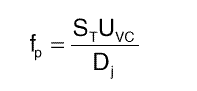The Strouhal experiments showed that the frequency of a single tone generated by turbulent flow is proportional to the fluid velocity and some characteristic dimension. The proportionality factor (ST) is known as the ‘Strouhal number." Since the standard is interested in the fluid noise generated by a jet through a nozzle opening, the fluid velocity used is the velocity at the vena contracta of the jet, and the characteristic dimension, of course, is the diameter of the jet. Further experiments have shown that the peak frequency (fp) of the "hay-stack" spectrum for control valves occurs at ST= 0.2.

The standard assumes that the noise level at the peak frequency is the most important and essentially ignores the noise level generated at other frequencies in the haystack spectrum. This, of course, is just a first level approximation which produces acceptable results. This approach can be justified by recalling that noise levels do not directly add to each other, but combine logarithmically. Thus, the peak noise level will tend to dominate with only minor contributions from the other frequencies. A correction factor is added later in the standard procedure to account for the contribution from these other frequencies.

# Calculating Transmission Loss

A direct calculation of transmission loss at any frequency is an extremely complicated affair. Dr. Fagerlund, in the work previously referenced, has developed an equation for the IEC standard which allows calculation of the transmission loss at the Ring Frequency. This calculation, used in conjunction with the transmission loss spectrum, allows one to extrapolate the Ring Frequency calculation to any other point on the curve. Since there are only three straight line regions in the transmission loss spectrum, this adjustment is a simple extrapolation using the slopes of the transmission loss spectrum and the distance along the frequency axis. Since most multi-hole noise

reduction trims accomplish much of their noise reduction by shifting the noise to a higher frequency, it is often the case that the noise peak frequency is located above the ring frequency as shown in Figure 5.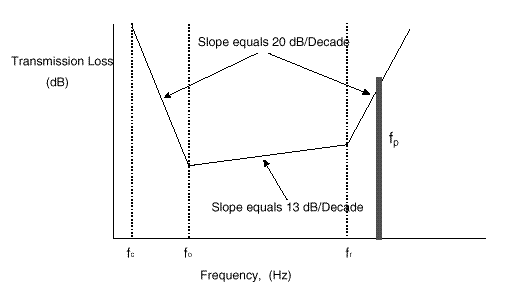Figure 5: Transmission Loss Calculation

It is obvious that this frequency shift produces additional trans-mission loss which reduces the external noise level, but it has the added advantage of placing the peak noise frequency above the normal range of human hearing where it is less offensive.

Once the transmission loss through the pipe has been calculated, this loss can then be subtracted from the sound pressure level inside the pipe. The result is the sound pressure level in the ambient air immediately outside the pipe wall. Using standard acoustic adjustment factors, this sound pressure level can be easily changed to a A-Weighting sound pressure level.

# Step 5: Translate Pressure Level toStandard Observer Location.

We can now conveniently think of the vibrating pipe wall as a new noise source. As the sound radiates outward from the pipe, which is essentially a line source, we can imagine a series of cylindrical wave front surfaces of ever increasing diameters. Figure 6 illustrates one such cylindrical wave front located at the observer’s location, one meter away from the outside of the pipe.Figure 6: Noise at the Observer Location

Since sound power level stays constant as it flows outward, the sound power level at each wave front surface is the same as any other, however, this power gets distributed over a larger and larger surface area as we get further and further from the source. This means that the amplitude of the pressure disturbance is decreasing with distance from the source. For a given length (L) of pipe, the surface areas of the pipe and one meter imaginary cylinder are respectively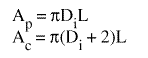These formulas give the surface areas of the respective cylinders at the surface of the pipe (A p and at the one meter distance (Ac).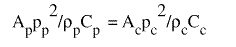Combining the sound pressure terms at each of these surfaces and using the sound power formula utilised previously, we can write an expression for the sound power at each of these surface areas.

Furthermore, we can logically assume that the air density (r) and the speed of sound (C ) are the same in the air at the external pipe wall and only one meter away at the observer. When these terms cancel, we see that the pressure disturbance at the observer location is simply the pressure disturbance at the pipe wall modified by the ratio of the areas involved. As a result, we can then finally calculate the Sound Pressure Level (SPL) at the observer location as a function of the known sound pressure level at the external pipe surface.

# Advantages of the IEC Standard

The IEC 534 noise prediction standard provides an accurate and objective approach to calculating aerodynamic control valve noise. The IEC standard is accepted worldwide. Some international companies require its use as a condition of purchase. The standard can be used for any manufacturer’s valves. Use of the IEC standard "levels the playing field," and allows purchasers of control valves to have an objective basis to com-pare competing offers.

1 A.C. Fagerlund & D.C. Chou, "Sound Transmission Through a Cylindrical Pipe Wall," Journal of Engineering for Industry,Vol. 103,November1981,pp355-360# Definitions

 A Flow passage area, m2 Ap Pipe surface area, m2 Ac Wave front surface area, m2 C Speed of sound, m/s Cp Speed of sound at pipe, m/s Cc Speed of sound at observer, m/s Di Internal pipe diameter, m Dj Vena contracta jet diameter, m fc Cutoff frequency, Hz fo First coincidence pipe frequency, Hz fr Ring frequency, Hz L Length of pipe section, m m Mass flow rate, kg/s Pd Sound pressure variation, Pa Po Reference sound pressure, 2x10-5Pa SPL Sound Pressure Level ST Strouhal number, Dimensionless Uvc Vena contracta velocity, m/s Wa Acoustic power, W Wm Stream power of mass flow, W hx Acoustic efficiency factor, Dimensionless r Fluid density, kg/m3 rp Fluid density at pipe, kg/m3 rc Fluid density at observer, kg/m3